Our Special Offer - Get 3 Courses at 24,999/- Only. Read more
Hire Talent (HR):+91-9707 240 250

# General# Bubble Sort in Python

Sorting refers to the data arrangement in an ascending or descending order based on the linear relationship across the elements. In this tutorial, we will learn about the Bubble Sort in Python clearly.

## What does Bubble sort refer to?

Bubble sort, otherwise knowns as sinking sort is a simple and easy to understand the algorithm that steps continuously on the list to be sorted, compare two adjacent items, and also swap them in case they are not in the right order. The steps tend to be repeated until they are arranged in the proper order as well when it reaches a situation where there are no swaps required. It is the place of a sorted list.

## How to perform a Bubble sort?

Here are the steps that can be followed to perform a bubble sort.

• You need to compare the element list, i.e. the first and second elements and swap them in the right order in case their arrangement is wrong.
• Similar to first, the same process needs to be executed for the second and third elements in the list. You need to swap them in the right order in case their arrangement is wrong.
• You need to follow the same until the last element in the list so that every element are arranged in the right way, and there is no need for swapping the elements.
• Keep repeating until the last list is arranged in the right order.

Let me explain this with the following visuals so that you will get a clear understanding of the bubble sort concept.

Visual 1: the initial element list## First Iteration:

Visual 2: We are going to compare the first two elements now.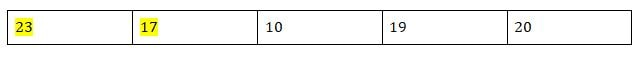As the first two elements are not arranged in the right format, we are going to swap them.

Visual 3: Swapping of the first two-element.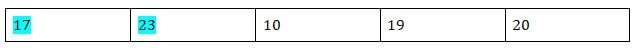Visual 4: compare the second and third elements now.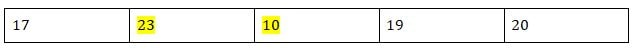Visual 5: Swapping of the second and third elements.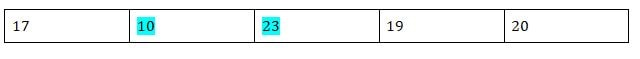Visual 6: compare the third and fourth elements now.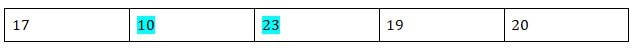Visual 7: Swapping of the third and fourth elements.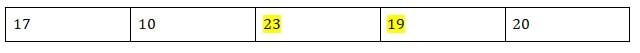Visual 8: compare the fourth and fifth elements now.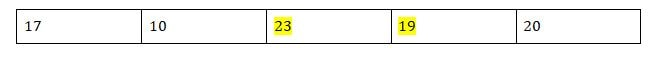Visual 9: Swapping of the fourth and fifth elements.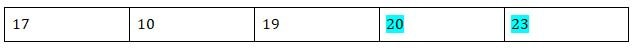## Second Iteration

Now we are going to follow the same as the first iteration.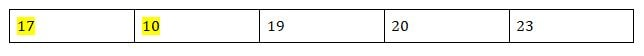### Swap: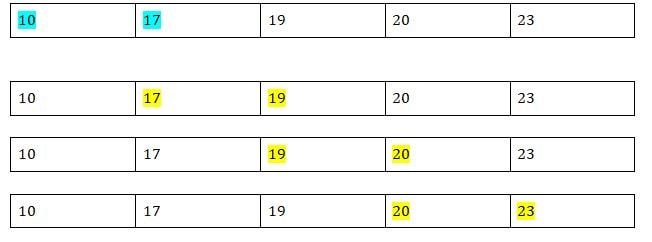## Third Iteration:

The same sorting is going to be performed similarly to the first and second iterations.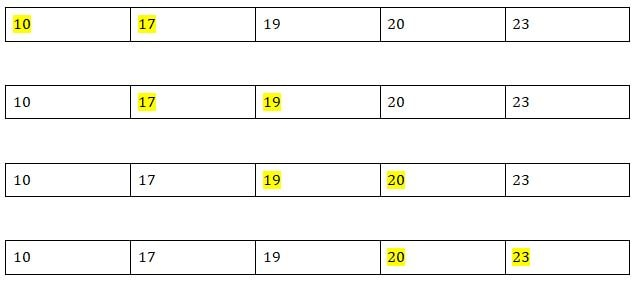As of now, everything is appropriately arranged, and therefore it will break the loop.

## Bubble Sort Algorithm

Let’s look after the algorithm behind this Bubble sort.

### First pass:

(23,17,10,19,20) → (17,23,10,19,20) – The algorithm compared the first and second element and swap them accordingly as 23>17

(17,23,10,19,20) → (17,10,23,19,20) – The algorithm compared the second and third element and swap them accordingly as 23>10

(17,10,23,19,20) → (17,10,19,23,20) – The algorithm compared the third and fourth element and swap them accordingly as 23>19

(17,10,19,23,20) → (17,10,19,20,23)- The algorithm compared the fourth and fifth element and swap them accordingly as 23>20

### Second pass:

(17,10,19,20,23) → (10,17,19,20,23) – The algorithm compared the first and second element and swap them accordingly as 17>10

(10,17,19,20,23) → (10,17,19,20,23) – Here the elements are arranged in the right order so no need to swap.

(10,17,19,20,23) → (10,17,19,20,23) – Here the elements are arranged in the right order so no need to swap.

(10,17,19,20,23) → (10,17,19,20,23) – Here the elements are arranged in the right order so no need to swap.

Now, we can see every array is sorted, but the algorithm will check again entirely as it’s developed to sort until there is no need for swapping. So the third iteration also occurs as the same.

### Third pass:

```(10,17,19,20,23) → (10,17,19,20,23)
(10,17,19,20,23) → (10,17,19,20,23)
(10,17,19,20,23) → (10,17,19,20,23)
(10,17,19,20,23) → (10,17,19,20,23)```

The final output will be (10,17,19,20,23).

Let’s check this with the program code.

## Python programming codes to implement bubble sort:

```x= [23,17,10,19,20]
#repeating loop len(x)(number of elements) number of times
for b in range(len(x)):
#initially swapped is false
swapped = False
a = 0
while a<len(x)-1: #comparing the adjacent elements if x[a]>x[a+1]:
#swapping
x[a],x[a+1] = x[a+1],x[i]
#Changing the value of swapped
swapped = True
a = a+1
#if swapped is false then the list is sorted
#we can stop the loop
if swapped == False:
break
print (x)```

The output of the above program will be

```[10,17,19,20,23]
Program finished with exit code 0
Press enter to exit the console.```

In the above code, you can see we are comparing the near numbers and then swapping them if they are not in the right arrangement. We have also repeated the same process len(x) number of times. We have assigned swapped as a variable and made is ‘True’ if the two elements are arranged in the right way. In case there is nothing to sort, then there will be no change of values, and then the loop can be broken.

I hope the above tutorial helped you to know the concept of Bubble sort in Python clearly. Any queries on the topic? Let us know through the comment section below.